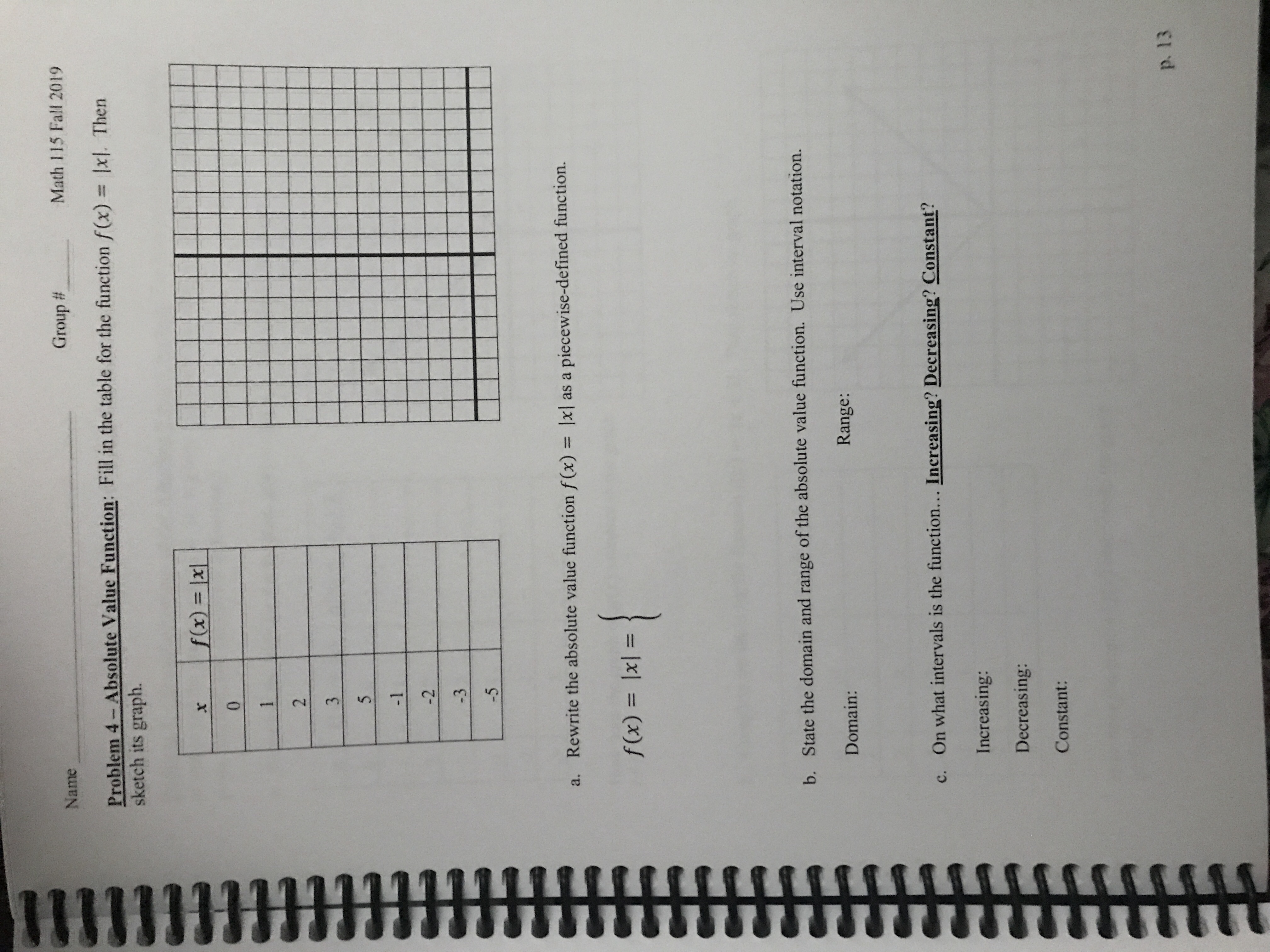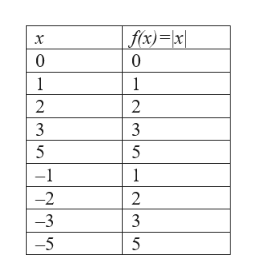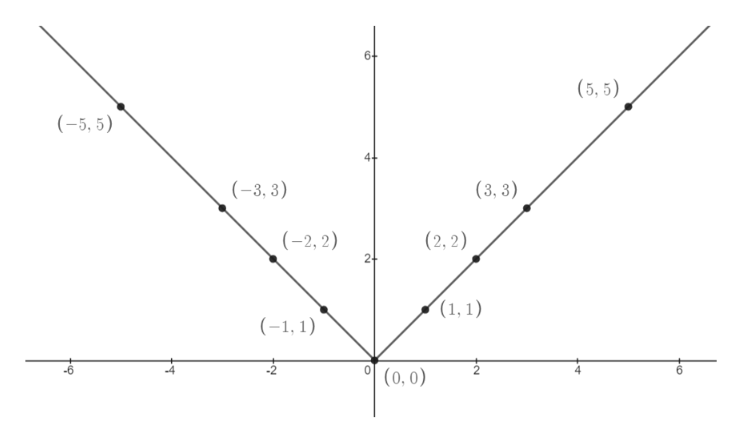# HtNameGroup #Math 115 Fall 2019Problem 4- Absolute Value Function: Fill in the table for the function f(x) = x1. Thensketch its graph.f(x) = |x|X01235-1-2-3-5a. Rewrite the absolute value function f(x) = |x||piecewise-defined function.as af(x) lxl =b. State the domain and range of the absolute value function. Use interval notation.Domain:Range:c. On what intervals is the function... Increasing? Decreasing? Constant?Increasing:Decreasing:Constant:p. 13

Question
9 viewshelp_outlineImage TranscriptioncloseHt Name Group # Math 115 Fall 2019 Problem 4- Absolute Value Function: Fill in the table for the function f(x) = x1. Then sketch its graph. f(x) = |x| X 0 1 2 3 5 -1 -2 -3 -5 a. Rewrite the absolute value function f(x) = |x|| piecewise-defined function. as a f(x) lxl = b. State the domain and range of the absolute value function. Use interval notation. Domain: Range: c. On what intervals is the function... Increasing? Decreasing? Constant? Increasing: Decreasing: Constant: p. 13 fullscreen
check_circle

Step 1

The given function is f(x) = |x|.

The table can be filled as follows.help_outlineImage Transcriptionclosef(x)=\x| x 0 0 1 2 3 3 5 5 -1 1 -2 2 -3 3 -5 5 2MTTT|| fullscreen
Step 2

Plot the function using the points from the table.help_outlineImage Transcriptionclose6 (5,5) (5, 5) (3, 3) (3, 3) (-2, 2) (2,2) (1,1) (-1, 1) (0, 0) fullscreen
Step 3

(a) The given function as piece...

### Want to see the full answer?

See Solution

#### Want to see this answer and more?

Solutions are written by subject experts who are available 24/7. Questions are typically answered within 1 hour.*

See Solution
*Response times may vary by subject and question.
Tagged in

### Functions